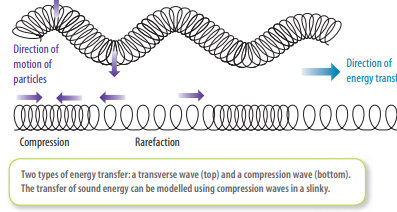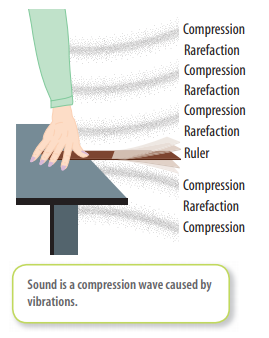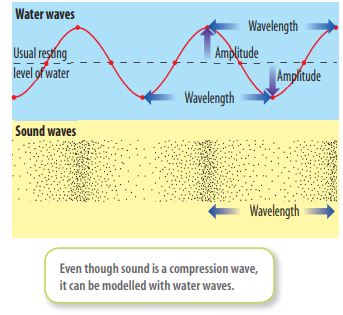Sangeetha Pulapaka
2

I assume you are referring to waves here. When a wave is made in a still lake by dropping a rock into it, the wave spreads out. However, the particles of water do not spread out — they just move up and down. A wave is able to transmit energy from one place to another without moving any matter over the same distance.

Waves on water are called transverse waves. Transverse waves can also be made on a slinky. As shown in the diagram below, the moving particles in a transverse wave travel at right angles to the direction of energy transfer.The diagram also shows that in a compression wave, the moving particles move backwards and forwards in the same direction as the  energy transfer. Compression waves are also known as longitudinal waves. The material through which the waves travel is called the medium (the plural is ‘media’).

Sound is a compression wave. All sounds are caused by vibrations. Vibrations cause air to compress like the lower wave pattern shown in the diagram below. The diagram above right shows how a vibrating ruler makes compression waves in air. As the ruler moves up, a compression is created as air particles above the ruler are pushed together. Air particles below the ruler are spread out, creating a rarefaction. When the ruler moves down, a rarefaction is created above the ruler, while a compression is created below it. Each vibration of the ruler creates new compressions and rarefactions to replace those that are moving through the air.Some jargon to learn :-

The frequency of a vibration or wave is the number of complete vibrations or waves made in one second. The frequency of a sound wave is given by the number of compressions made in a second. The note middle C, for example, creates 256 vibrations, or compressions, every second. Frequency is measured in hertz (Hz), a unit named after Heinrich Hertz, the German physicist who, in 1887, was the fi rst to detect radio waves. One hertz is equal to one vibration per second. Therefore, middle C has a frequency of 256 hertz. The frequency of a sound determines its pitch. Highfrequency vibrations produce high pitch, and low-frequency vibrations produce low pitch. In the case of transverse waves, for example waves on water, the wavelength is the distance between two crests, or two troughs, or the distance between any two corresponding points on neighbouring waves. In the case of a compression wave, the wavelength is the distance between the centre of two neighbouring compressions, ortwo neighbouring rarefactions, as shown in the diagram below. The distance between compressions — the wavelength — of the sound of the note middle C is about 1.3 metres. The wavelength of sound made during normal speech varies between about 5 centimetres and about 2.5 metres. As the frequency of a sound gets higher — that is, as more compressions are produced per second — the compressions become closer together.The pitch you hear is higher. Lower frequencies produce longer wavelengths and thus lower-pitched sounds. The amplitude of a wave is the maximum distance that each particle moves away from its usual resting position. The amplitude of two different types of waves is also shown in the diagram below. Higher amplitudes correspond with louder sounds.